# The Effect of Bluff Body Shape on Flame Stability in a Non-Premixed Hydrogen-Methan-Air Mixture Combustion

The Effect of Bluff Body Shape on Flame Stability in a Non-Premixed Hydrogen-Methan-Air Mixture Combustion

Fatma Zohra Khelladi Mounir Alliche Redha Rebhi Giulio LorenziniHijaz Ahmad Younes Menni

Laboratoire de Transports Polyphasiques et Milieux Poreux (LTPMP), Université de Technologies Houari Boumediene, Bab Ezzouar, Algiers, Algeria

Department of Mechanical Engineering, Faculty of Technology, University of Medea, Medea 26000, Algeria

LERM-Renewable Energy and Materials Laboratory, University of Medea, Medea 26000, Algeria

Department of Engineering and Architecture, University of Parma, Parco Area delle Scienze, 181/A, Parma 43124, Italy

Department of Basic Science, University of Engineering and Technology, Peshawar 25000, Pakistan

Department of Technology, University Center Salhi Ahmed Naama (Ctr. Univ. Naama), P.O. Box 66, Naama 45000, Algeria

Corresponding Author Email:
Giulio.lorenzini@unipr.it
Page:
385-392
|
DOI:
https://doi.org/10.18280/acsm.450504
23 April 2021
|
Accepted:
16 July 2021
|
Published:
31 October 2021
| Citation

OPEN ACCESS

Abstract:

The goal of this study, which focuses on the effect of the bluff-body form on the flame’s stability, is to contribute to the study of the stability of a CH4-H2-Air diffusion flame. It is, in fact, a numerical simulation of a diffusion flame CH4-H2-Air stabilized by a bluff body in three different shapes: cylindrical, semi-spherical and conical. The equations governing turbulent reactive flow are solved using the Ansys CFX program (Navier Stokes equations averaged in sense of Favre). The k-ε model simulates turbulence. For combustion, a mixed EDM/FRC (Finite Rate Combustion) model is utilized. The results of the analysis of temperature profiles, CO2 concentrations, and velocity in axial sections very close to the injector are satisfactory: they meet the criteria of stability, high temperature at a lower speed, and more stable in the case of a cylindrical shape than in the other two cases.

Keywords:

CH4-H2-Air mixture, CO2 concentrations, non-premixed combustion, finite rate combustion, stabilization, flame stability, bluff body

1. Introduction

The principle of stabilization of a flame can be summarized in the existence of a high temperature zone associated with a zone of slowdown of speed, in order to create a continuous thermal source able to maintain the combustion. This requires, on the one hand, an equal flow velocity and propagation zone of the flame and, on the other hand, a sufficiently long contact time for the mixing to take place. Thus, several practical devices can be used to stabilize a flame: a pilot flame, an obstacle (bluff-body), a plate or a bar placed in the flow mixture, and as often used in industry, a recirculation zone with a swirl . Many authors confirm that the use of a bluff-body type burner is undoubtedly the most interesting technique for the stabilization of a non-premixed flame, according to Nguyen . Due to their relevance to several practical applications, such as bluff-body combustors, which are widely used in industrial applications , bluff-body flames have received much interest in recent times because of their better overall mixing properties, enhanced flame stability, and ease of combustion control. In bluff-body stabilized flames, a vortex around the bluff body creates a complicated turbulent environment similar to that observed in industrial combustors. These flames, on the other hand, have simple and well-defined boundary requirements. As a result, bluff-body stabilized flames are an excellent model for investigating the interaction of turbulence and chemical reaction, and they may be used to bridge the gap between theoretical issues and engineering applications . A noteworthy model issue is a bluff-body stabilized jet diffusion flame, which can be used to a wide range of engineering combustion systems . The stabilization of a flame by a bluff-body is a process whose principle is to place in the flow a hurdle type 'bluff-body', which may be the lips of the burner, to allow the development of the recirculation zones (hot zone, stable, associated with a slowdown zone) in its wake . Several practical and theoretical research have been conducted on this flame stabilization approach. Correa et al.  used laser Raman scattering and the joint velocity-composition probability density function model to analyze a stable non-premixed methane-air flame. They discovered that without the use of a pilot flame, the bluff-body burner creates a very turbulent field leads to localized extinction, and that the recirculation stabilized flame investigated was considerably closer to real burners. Dally et al.  took measurements in the well-defined vortex, behind the axisymmetric bluff-body reacting methane flame, and compared the results to the results of a numerical simulation employing the conventional and modified k-epsilon type turbulence techniques. The bluff-body recirculation zone exhibited two distinct structures, according to their findings: a small inner vortex closest to the jet and a big outer vortex close to the air side. Dally and Masri  have demonstrated that flames stabilized on both piloted and bluff-body burners are comparable by calculating differential diffusion parameters from experimental data in non-premixed flames in turbulence of various fuel mixes and Reynolds numbers. In an experimental setup, Ahmed et al.  built a novel turbulent non-premixed bluff-body burner with radial fuel injection to study the spark ignition characteristics in a complicated design applicable to gas turbine combustors. The stability limits are substantially broader than the ignitability limits, but they get closer with swirl, and that the mixture fraction distribution is extremely sensitive to variations in fuel and air velocities, with the mixture inside the vortex tending to be well-mixed and rich. With biogas as fuel, Noor et al.  investigated the vortex and ignition site for a bluff-body non-premixed MILD burner. According to their calculations, the inner vortex between the air and fuel nozzle is the best location for the ignition point since the low velocity of air and fuel mixing will enhance the igniting process. The ignition energy will have ample time to ignite the combination due to the low speed turbulent swirl flow. In addition, to the computational research, Dally et al.  provided a comprehensive collection of experimental data with variable fuel jet velocities exhibiting variable amounts of local extinction. The flames are labeled as HM1, HM2 or HM3 depending on the fuel jet velocity. In the turbulent combustion community, Sydney bluff-body flames have long been a favored target flame. As a results, numerous researchers have examined the HM1 flame numerically, using a variety of fuels, models, and methodologies. Muradoglu et al.  used a combined velocity and turbulent PDF technique for frequency compositions combined with a coherent particle/hybrid solution technique to estimate the performance of the combined PDF method in terms of flow prediction and standard mean fields by comparing the calculated radial plots with the available experimental data. Kim and Pitsch  used large turbulent eddy vortex simulations and an integrated formulation of a conditional filtration technique to examine the dynamics of flow, standard mixing, and production in the same flame at sub-filter sizes. They observed that there is a huge numerical dissipation and intensive mixing of the fuel and air near the end of the recirculation zone, when turbulence is completely established. In two separate incidents, Hossain and Malalasekera [11, 12] worked on the Sydney flame. They used a laminar flamelet model and a k-turbulence model to examine the structure of the field, flame structure, mixing pattern, and turbulence fluctuations in the first scenario , which included both HM1and HM3 flames. They examined three distinct areas of the deceptive body flame: (i) recirculation, (ii) neck, and (iii) puff-like region. Inside the recirculation zone, two vortices were discovered. For the HM1 flame, there is a larger outer vortex on the bluff-face, body’s and a much smaller inner vortex further downstream. The inner vortex loses its recirculation pattern and combines with the jetting in an HM3 flame, while the outer vortex decreases and becomes shorter. They next looked at the HM1 flame in the second scenario  to see how well the various combustion models predicted pre-mixed turbulent combustion when combined with the k-turbulence model. Sheet flame models, equilibrium chemistry, finite equilibrium, and laminar flamelet were utilized to simulate combustion in this flame.

However, few research on the effect of bluff-body from in non-premixed combustion have been conducted. An experimental study of the influence of the geometry of the stabilizer on the development of a non-premixed flame made by Nguyen  showed that the recirculation zone generated by a disk stabilizer, was longer, wider and more intense than that observed in the case of the tulip, that the aerodynamics of the return flow generated by the disk was more disturbing than that created by the tulip and that the stabilization domain is more extensive in the case of a wake of smaller size where the recirculation speeds are lower, that is to say in the case of the tulip rather than that of the disc. Based on this last remark and using a tulip stabilizer, Esquiva-Dano and Escudie  carried out another experimental study on a non-premixed flame stabilized by the shaped obstacle (tulip) to present five stabilization regimes. Among them, the triple flame regime or the blue ring flame whose stabilization point was located at the interface between the recirculation zone in the wake, consisting of a premix of fuel, air and products combustion, and the annular air flow. Devaraj and Maran  looked into the influence of illusion body form and discovered that combustion with a solid cylindrical illusion body produces temperatures twice as high as combustion with a circlip, but with a substantially bigger pressure drop, which is the major drag driver. Other studies are also important and have dealt with various problems of combustion in different media such as Ren et al. , Felsch , Lemay , Zohra et al. , Ma et al. , Rakopoulos et al. , Liu and Reitz , Mohammed et al. , Rakopoulos et al. , Juntarakod et al. , Olikara and Borman , etc.

In this context and using the HM1 flame, the present study aims to determine the influence of the shape of the bluff-body on the stabilization of a CH4-H2-Air diffusion flame whose fuel mixture is equal to the volume fraction of methane and hydrogen (50:50 CH4 / H2). To do this, we dared to modify the 'head' of the bluff-body (the nozzle of the injector acting as an obstacle). A numerical simulation of this flame was carried out using three forms of bluff-body: cylindrical, conical and semi-spherical.

2. Materials and Methods

The geometry employed by Ren  has been applied in this investigation, with the same fuel and air input conditions; it is a flame (HM1 flame) whose fuel, methane-hydrogen, is surrounded by a bluff-body, which is surrounding by a co-current of air. Figure 1 depicts the computational domain, as proposed in the linked study . The center of the plane of the jet outlet is used as the coordinate system’s origin.

## 1.pngFigure 1. Schematic drawing of the computational domain

The following numerical circumstances were chosen for the calculation:

• A diameter of 0.05 m for the bluff-body known as a Sydney bluff-body, Db;
• An axial velocity of 118 m/s for the fuel, with a turbulence intensity of 10% and a hydraulic diameter of 0.0036 m, at a temperature of 300 K; and
• At a temperature of 300 K, an air co-current of 40 m/s with a turbulence intensity of 10% and a hydraulic diameter of 0.25 m.

The equations governing reactive turbulent flow are solved using the ANSYS CFX program (Navier Stokes equations averaged in sense of Favre). The k-ε model is used to simulate turbulence. For combustion, the combined EDM/FRC (Eddy Dissipation Model/Finite Rate Combustion) model is utilized. With constant transport parameters, the flow calculation code solves the conservation equations for mass, velocity, turbulence, energy, and species. According to Felsch , the set of equations governing the flow is described in this publication. However, we solely consider the stationary scenario in our research.

The entire mass conservation equation is as follows:

$\frac{\partial \bar{\rho}}{\partial t}+\frac{\partial}{\partial x_{i}}\left(\bar{\rho} \tilde{u}_{i}\right)=0$    (1)

The mass fractions of the species are calculated using the following equations:

$\frac{\partial}{\partial t}\left(\bar{\rho} \tilde{Y}_{n}\right)+\frac{\partial}{\partial x_{i}}\left(\bar{\rho} \tilde{Y}_{n} \tilde{u}_{i}\right)=\frac{\partial}{\partial x_{i}}\left[\left(\frac{\mu}{S_{c}}+\frac{\mu_{t}}{S_{c, t}}\right) \frac{\partial \tilde{Y}_{n}}{\partial x_{i}}\right]+\bar{\rho} \widetilde{\omega}_{n}$    (2)

The principle of momentum conservation:

$\frac{\partial}{\partial \mathrm{t}}\left(\bar{\rho} \tilde{u}_{i}\right)+\frac{\partial}{\partial \mathrm{x}_{j}}\left(\bar{\rho} \tilde{u}_{i} \tilde{u}_{j}\right)=-\frac{\partial \bar{p}}{\partial x_{i}}$

$+\frac{\partial}{\partial \mathrm{x}_{\mathrm{j}}}\left[\mu\left(\frac{\partial \tilde{u}_{i}}{\partial \mathrm{x}_{\mathrm{j}}}+\frac{\partial \tilde{u}_{j}}{\partial \mathrm{x}_{\mathrm{i}}}-\frac{2}{3} \frac{\partial \tilde{u}_{l}}{\partial x_{l}} \delta_{\mathrm{ij}}\right)-\tilde{\rho} \widetilde{\mathrm{u}_{\mathrm{i}}^{\prime \prime} \mathrm{u}_{\mathrm{j}}^{\prime \prime}}\right]$    (3)

The Reynolds stresses are the terms $\tilde{\rho} \widetilde{\mathrm{u}_{\mathrm{i}}^{\prime \prime} \mathrm{u}_{\mathrm{j}}^{\prime \prime}}$. The classic turbulence, k-epsilon, model is used to model them. Gravity and body forces are not taken into account.

The standard k-ε model is an approximate model or strategy for resolving governing equations in which unknown terms, such as Reynolds stresses, arise. As a result, certain assumptions have been made.

$\tilde{\rho} \widetilde{\mathrm{u}_{\mathrm{i}}^{\prime \prime} \mathrm{u}_{\mathrm{j}}^{\prime \prime}}=\mu_{t}\left(\frac{\partial \tilde{u}_{i}}{\partial x_{j}}+\frac{\partial \tilde{u}_{j}}{\partial x_{i}}\right)-\left(\frac{2}{3} \bar{\rho} \tilde{k}+\frac{2}{3} \mu_{t} \frac{\partial \tilde{u}_{l}}{\partial x_{l}}\right)$    (4)

With $\mu_{t}=C_{\mu} \bar{\rho} \frac{\tilde{k}^{2}}{\tilde{\varepsilon}}$. If the values for $\tilde{k}$ and $\tilde{\varepsilon}$ are known, the Reynolds stresses may be calculated. This leads to the k-ε model, which solves the model transport equations for $\tilde{k}$ and $\tilde{\varepsilon}$. The kinetic energy of turbulence is calculated using the following equation:

$\frac{\partial}{\partial \mathrm{t}}(\bar{\rho} \tilde{\mathrm{k}})+\frac{\partial}{\partial \mathrm{x}_{\mathrm{i}}}\left(\bar{\rho} \tilde{\mathrm{u}}_{\mathrm{i}} \tilde{\mathrm{k}}\right)=\frac{\partial}{\partial \mathrm{x}_{\mathrm{i}}}\left[\left(\mu+\frac{\mu_{\mathrm{t}}}{\sigma_{\mathrm{k}}}\right) \frac{\partial \tilde{\mathrm{k}}}{\partial \mathrm{x}_{\mathrm{i}}}\right]+\bar{\rho}\left(\mathrm{P}_{\mathrm{k}}-\tilde{\varepsilon}\right)$    (5)

Pk is the k production, calculated as follows:

$P_{k}=\frac{\mu_{t}}{\tilde{\rho}}\left(\frac{\partial \tilde{u}_{i}}{\partial \mathrm{x}_{\mathrm{j}}}+\frac{\partial \tilde{u}_{j}}{\partial \mathrm{x}_{\mathrm{i}}}\right) \frac{\partial \tilde{u}_{i}}{\partial x_{j}}-\frac{2}{3}\left(\tilde{k}+\frac{\mu_{t}}{\bar{\rho}} \frac{\partial \tilde{u}_{l}}{\partial x_{l}}\right) \frac{\partial \tilde{u}_{i}}{\partial x_{i}}$    (6)

Finally, the following equation  is used to estimate the rate of dissipation of k:

$\frac{\partial}{\partial t}(\bar{\rho} \tilde{\varepsilon})+\frac{\partial}{\partial x_{i}}\left(\bar{\rho} \tilde{u}_{i} \tilde{\varepsilon}\right)=\frac{\partial}{\partial x_{i}}\left[\left(\mu+\frac{\mu_{t}}{\sigma_{\varepsilon}}\right) \frac{\partial \tilde{\varepsilon}}{\partial x_{i}}\right]+\bar{\rho} \frac{\tilde{\varepsilon}}{\tilde{k}}\left(C_{\varepsilon 1} P_{k}-C_{\varepsilon 2} \tilde{\varepsilon}\right)$    (7)

The values of five constants of the standard k-epsilon (Cμ, σk, σε, Cε1 and Cε2) are determined from an experiment for a shear flow investigation.

Energy conservation entails:

$\frac{\partial}{\partial t}\left(\bar{\rho} \widetilde{e}_{t}\right)+\frac{\partial}{\partial x_{i}}\left(\bar{\rho} \tilde{e}_{t} \tilde{u}_{i}\right)=\frac{\partial}{\partial x_{i}}\left[\left(\frac{\mu}{P_{r}}+\frac{\mu_{t}}{P_{r, t}}\right) \frac{\partial \tilde{e}_{t}}{\partial x_{i}}\right]-$

$\frac{\partial}{\partial \mathrm{x}_{\mathrm{i}}}\left[\frac{\mu}{\sigma} \frac{\partial}{\partial \mathrm{x}_{\mathrm{i}}}\left(\frac{1}{2} \widetilde{\mathrm{u}}_{\mathrm{j}} \tilde{\mathrm{u}}_{\mathrm{j}}+\tilde{\mathrm{k}}-\widetilde{\mathrm{R}} \widetilde{\mathrm{T}}\right)\right] \frac{\partial}{\partial \mathrm{x}_{\mathrm{i}}}\left(\bar{\rho} \tilde{\mathrm{u}}_{\mathrm{i}}\right)+\frac{\partial}{\partial \mathrm{x}_{\mathrm{i}}}\left(\bar{\tau}_{\mathrm{ji}} \tilde{\mathrm{u}}_{\mathrm{j}}\right)$    (8)

The computational domain was discretized using the same mesh; a multizone mesh with 49,474 nodes for 45,379 elements in the cylindrical bluff body, 40,771 nodes for 45,648 elements in the semi-spherical bluff body, and 40,093 nodes for 45,268 elements in the conical bluff body, which required about 3,000 iterations.

3. Results and Discussion

The results of our calculation are presented in terms of temperature, velocity and CO2 mass fraction profiles. The analysis of these results in axial sections downstream of the injector and in the vicinity of the latter, and more precisely in the recirculation zone (0.26 < Y/Db < 1.3) and in the mixing zone (1.3 < Y/Db < 1.8) as defined by some studies [9, 17] shows that the cylindrical shape of the bluff body satisfies the principle of stabilization of a flame which can be summarized, according to Kim and Pitsch , to the existence of a zone at high temperature, associated with a slowing zone of speed, in order to create a source continuous thermal capable of maintaining combustion.

To ensure the convergence of the multi-zone mesh, we firstly tested its sensitivity on different calculation grids with an increasing number of cells (Table 1), all having a non-uniform multi-zone mesh.

Table 1. Number of mesh cells and nodes

 Grid Cells Nodes 1 26,475 29,262 2 45,379 49,474 3 65,024 70,158

In order to assess the grid sensitivity of the results presented, additional calculations on a progressive refined grid were carried out. In each case, the grid was obtained by multiplying the number of points by a factor of 1.2, in both x and y directions. The comparison between the results obtained with coarse (26,475 cells), fine (45,379 cells) and dense (65,024 cells) grid, is reported in Figures 2 and 3. It can be shown that, in terms of radial velocity and temperature profiles, the solution convergence has been reached and the solution becomes independent of the mesh sizes over the mesh called fine mesh. Further mesh refinement was tested and not presented for brevity.

## 2a.png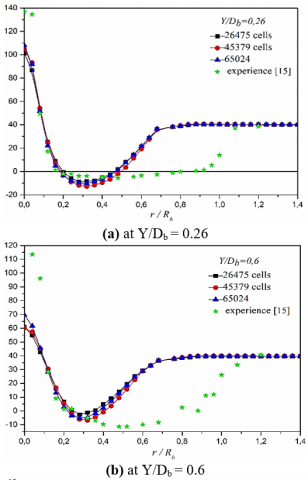## 2b.png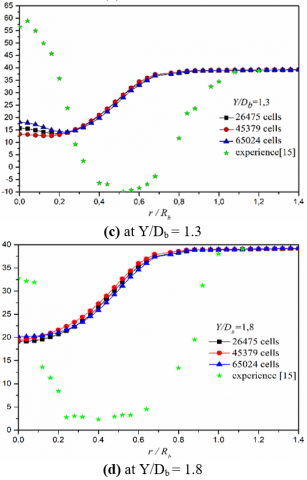Figure 2. Radial velocity profile for different mesh grids in various flow sections

Figures 4, 5 and 6 show the structure of the flame for each bluff-body shape. To begin with, it demonstrates that this structure is dependent on the bluff body shape, and that the flame does not flourish as much in the case of a conical or semi-spherical bluff body as it does in the case of a cylindrical bluff body. In the case of a cylindrical bluff body, the maximum temperature of the flame is substantially higher than in the other two cases: 1973 K versus 1529 K in the conical case and 1457 K in the semi-spherical case (Figure 4).

## 3a.png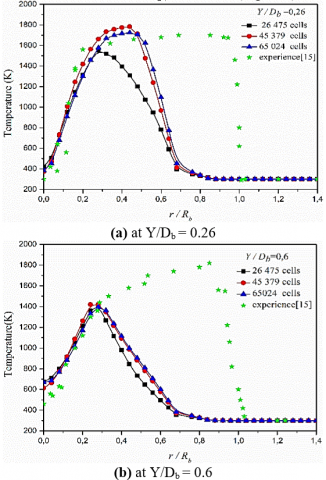## 3b.pngFigure 3. Radial temperature profile for different mesh grids in various flow sections

## 4.png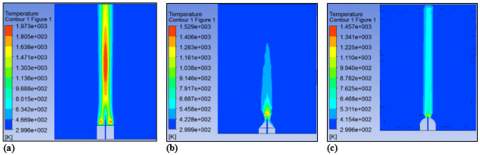Figure 4. Temperature iso-contours for different bluff body shape: (a) cylindrical, (b) conical and (c) semi-spherical bluff bodies

## 5.png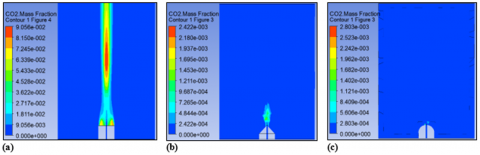Figure 5. CO2 mass fraction iso-contours for different bluff body shape: (a) cylindrical, (b) conical and (c) semi-spherical bluff bodies

## 6.png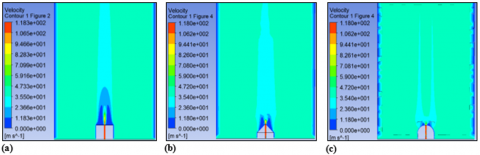Figure 6. Velocity iso-contours for different bluff body shape: (a) cylindrical, (b) conical and (c) semi-spherical bluff bodies

## 7.png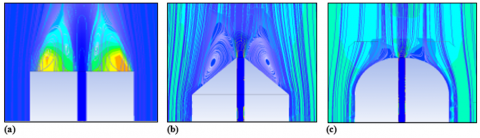Figure 7. Effect of the bluff-body shape on the wake structure for different types of mesh

The highest mass fraction of CO2 in a semi-spherical and even conical bluff body is lower than in a cylindrical bluff body; 0.13 % in the semi-spherical case, 0.24 % in the conical case, vs 9.05 % in the cylindrical case. It’s worth noting that, in comparison to the cylindrical shape, the evolution of the mass fraction of CO2 is low in these latter two shapes (Figure 5).

In the two cases of spherical and conical forms of the bluff-body, there is no discernible difference in the flame propagation speed (Figure 6).

Figure 7 depicts the streamlines near the injector’s outflow (the wake structure). The shape of the wake that forms downstream of the bluff body and serves as a recirculation and mixing zone is determined by the bluff body’s shape. In the event of a semi-spherical shape of the barrier, the vortices created at the injector outlet are quite small, which decreases the mixture and trends to and blow out the flame. These vortices are larger and tend to bypass the flame in the case of a conical obstruction, smothering the fame. The results also demonstrate that these current lines are uniform of oval forms concentric in the cylindrical shape a little further from the injector, that is, in the zone of propagation of the jet, whereas in the two other situations, these current lines scatter, tend to break, and their shape cannot be determined.

To examine the flame stability in each case, Figure 8 highlights the flame stability criterion, which can be summarized as the existence of a zone of high temperature, associated with a slowing zone of speed, in order to create a continuous thermal source capable of maintaining combustion, according to Kim and Pitsch . Indeed, the evolution of temperature is more critical in the case of a cylindrical-shaped bluff body than in the other two scenarios for distinct axial sections near the injector (recirculation zone and mixing zone).

## 8a.png## 8b.png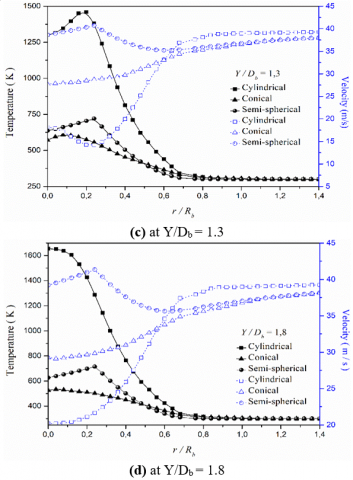Figure 8. Radial temperature/velocity profiles at different axial location for different bluff body shape: temperature in solid line / velocity in dashed line

The radial evolution of temperature in axial parts extremely close to the injector is higher in the case of a cylindrical bluff body than in the other two scenarios. It’s worth noting that the temperature radial evolution in the semi-spherical shape of the bluff body is slightly higher than in the conical shape. In terms of speed, it declines radially before increasing and stabilizing at roughly 40 m/s (air velocity). We should also remark that the bluff of a cylindrical body has the lowest speed before reaching the zone of continuity.

Basing on these findings, we may conclude that the bluff-cylindrical body’s shape fits the flame stability criteria: the presence of a high temperature zone associated with a zone of decrease in speed, in order to establish a continuous heat source capable of maintaining combustion.

## 9a.png## 9b.pngFigure 9. Radial CO2 mass fraction profiles for different bluff body shape

Figure 9 indicates that the variation in CO2 mass fraction is small (nearly zero) in the case of a conical or semi-spherical bluff-body shape and in areas close to the injector outlet as compared to a cylindrical bluff-body shape. Incomplete combustion induced by smothering or blowing of the flame could explain the reduced or almost negligible carbon dioxide mass fraction in both the conical and semi-spherical forms.

4. Conclusions

In this paper, to examine the effect of the bluff-body shape on the stabilization of a non-premixed hydrogen-methane-air combustion, a numerical simulation was run using the Ansys CFX program. The following are the major conclusions:

• The stability of the flame, in this example a methane-hydrogen-air flame, is influenced by the shape of bluff-body.
• The cylindrical form is the only one of the three types of obstacles analyzed (non-contoured obstacles) that fits the stability requirement.
• In the case of a cylindrical shape of the bluff body, the maximum temperature of the flame is substantially higher than in the other two examples.
• In the case of a semi-spherical and even conical shape of the bluff body, the maximum mass fraction of CO2 is lower than in the case of a cylindrical shape of the bluff body: 0.13% in the semi-spherical case, 0.24% in the conical case against, vs 9.05% in the cylindrical case.
• In comparison to the cylindrical shape, the evolution of CO2 mass fraction is negligible in these latter two forms.
• In the event of a semi-spherical obstruction, the vortices created at the injector’s output are quite small, reducing the mixture and threatening to extinguish the flame. These vortices are larger and tend to bypass the flame in the case of a conical obstruction, smothering the flame.
• The cylindrical shape of the bluff-body satisfies the flame stability criteria, which necessitate a high temperature zone in conjunction with a slow sped zone in order to provide a continuous thermal source capable of maintaining combustion.

In overall, the current study shows that using bluff-bodies in combustors is a viable technique for improving heat transfer and flame stabilization.

References

 Nguyêñ, H.T. (1999). Etude expérimentale de l'influence de la géométrie de stabilisateur sur le développement d'une flamme non prémélangée. Doctoral dissertation, Ecully, Ecole centrale de Lyon.

 Muradoglu, M., Liu, K., Pope, S.B. (2003). PDF modeling of a bluff-body stabilized turbulent flame. Combustion and Flame, 132(1-2): 115-137. https://doi.org/10.1016/S0010-2180(02)00430-3

 Liu, K., Pope, S.B., Caughey, D.A. (2005). Calculations of bluff-body stabilized flames using a joint probability density function model with detailed chemistry. Combustion and Flame, 141(1-2): 89-117. https://doi.org/10.1016/j.combustflame.2004.12.018

 Kim, S.H., Pitsch, H. (2006). Mixing characteristics and structure of a turbulent jet diffusion flame stabilized on a bluff-body. Physics of Fluids, 18(7): 075103. https://doi.org/10.1063/1.2221352

 Correa, S.M., Gulati, A., Pope, S.B. (1994). Raman measurements and joint PDF modeling of a nonpremixed bluff-body-stabilized methane flame. In Symposium (International) on Combustion, 25(1): 1167-1173. https://doi.org/10.1016/S0082-0784(06)80755-1

 Dally, B.B., Masri, A.R., Fletcher, D.F. (1995). Modelling of bluff-body recirculating flows. In Twelfth Australasian Fluid Mechanics Conference, pp. 529-532.

 Dally, B.B., Masri, A.R. (1998). Measurements of differential diffusion in turbulent non premixed Flames. In 13th Australasian Fluid Mechanics Conference, Monash University, Melbourne, 13-18, Australia.

 Ahmed, S.F., Balachandran, R., Marchione, T., Mastorakos, E. (2007). Spark ignition of turbulent non premixed bluff-body flames. Combustion and Flame, 151(1-2): 366-385. https://doi.org/10.1016/j.combustflame.2007.06.012

 Noor, M.M., Wandel, A.P., Yusaf, T. (2013). Analysis of recirculation zone and ignition position of non-premixed bluff-body for biogas MILD combustion. International Journal of Automotive and Mechanical Engineering, 8(1): 1176-1186.

 Dally, B.B., Masri, A.R., Barlow, R.S., Fiechtner, G.J. (1998). Instantaneous and mean compositional structure of bluff-body stabilized non premixed flames. Combustion and Flame, 114(1-2): 119-148. https://doi.org/10.1016/S0010-2180(97)00280-0

 Hossain, M., Malalasekera, W. (2005). Numerical study of bluff-body non-premixed flame structures using laminar flamelet model. Proceedings of the Institution of Mechanical Engineers, Part A: Journal of Power and Energy, 219(5): 361-370. https://doi.org/10.1243/095765005X28616

 Hossain, M., Malalasekera, W. (2007). A combustion model sensitivity study for CH4/H2 bluff-body stabilized flame. Proceedings of the Institution of Mechanical Engineers, Part C: Journal of Mechanical Engineering Science, 221(11): 1377-1390. https://doi.org/10.1243/09544062JMES336

 Esquiva-Dano, I, Escudie, D. (2001). Effet de la turbulence sur la stabilisation d’une flamme non-prémélangée. In XVème Congrès Français de Mécanique Nancy, 3-7.

 Devaraj, P.R., Maran, P. (2014). Effects of inlet parameters on the performance of bluff body combustion. International Journal of Innovative Research in Science, Engineering and Technology, 3(6): 9.

 Ren, Z., Goldin, G.M., Hiremath, V., Pope, S.B. (2013) Simulations of a turbulent non-premixed flame using combined dimension reduction and tabulation for combustion chemistry. Fuel, 105: 636-644. https://doi.org/10.1016/j.fuel.2012.08.018

 Felsch, C. (2003). Investigation of boundary conditions and pressure waves in numerical modelling of compressible turbulent flow with combustion. Thèse de Doctorat, Trondheim, Norwegian University of Science and Technology.

 Lemay, J. (2010). Étude numérique des Conditions aux limites en situation de combustion turbulente non-prémélangée. Mémoire de Maîtrise en Génie Mécanique M. ING., Ecole de Technologie Supérieure Université du Québec, Montréal.

 Zohra, K.F., Mounir, A., Salah, C. (2017). Numerical simulation of CH4-H2-Air non-premixed flame stabilized by a bluff body. Energy Procedia, 139: 530-536. https://doi.org/10.1016/j.egypro.2017.11.249

 Ma, T., Feng, L., Wang, H., Liu, H.F., Yao, M.F. (2019). Analysis of near wall combustion and pollutant migration after spray impingement. International Journal of Heat and Mass Transfer, 141: 569-579. https://doi.org/10.1016/j.ijheatmasstransfer.2019.07.001

 Rakopoulos, D.C., Rakopoulos, C.D., Giakoumis, E.G., Papagiannakis, R.G. (2018). Evaluating oxygenated fuel’s influence on combustion and emissions in diesel engines using a two-zone combustion model. Journal of Energy Engineering, 144(4): 04018046. https://doi.org/10.1061/(ASCE)EY.1943-7897.0000556

 Liu, Y., Reitz, R.D. (1998). Modeling of heat conduction within chamber walls for multidimensional internal combustion engine simulations. International Journal of Heat and Mass Transfer, 41(6): 859-869. https://doi.org/10.1016/S0017-9310(97)00178-6

 Mohammed, S.E., Baharom, M.B., A. Aziz, A.R., Zainal A., Ezrann, Z. (2019). Modelling of combustion characteristics of a single curved-cylinder spark-ignition crank-rocker engine. Energies, 12(17): 3313. https://doi.org/10.3390/en12173313

 Rakopoulos, C.D., Rakopoulos, D.C., Kyritsis, D.C. (2003). Development and validation of a comprehensive two-zone model for combustion and emissions formation in a DI diesel engine. International Journal of Energy Research, 27(14): 1221-1249. https://doi.org/10.1002/er.939

 Juntarakod, P., Soontornchainacksaeng, T. (2014). A quasi-dimensional three-zone combustion model of the diesel engine to calculate performances and emission using the diesel-ethanol dual fuel. Contemporary Engineering Sciences, 7(1): 19-37. http://dx.doi.org/10.12988/ces.2014.3951

 Olikara, C., Borman, G.L. (1975). A computer program for calculating properties of equilibrium combustion products with some applications to IC engines. SAE Technical Paper. https://doi.org/10.4271/750468